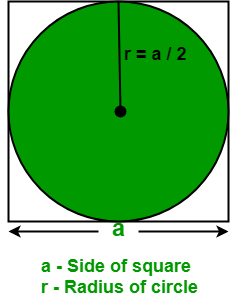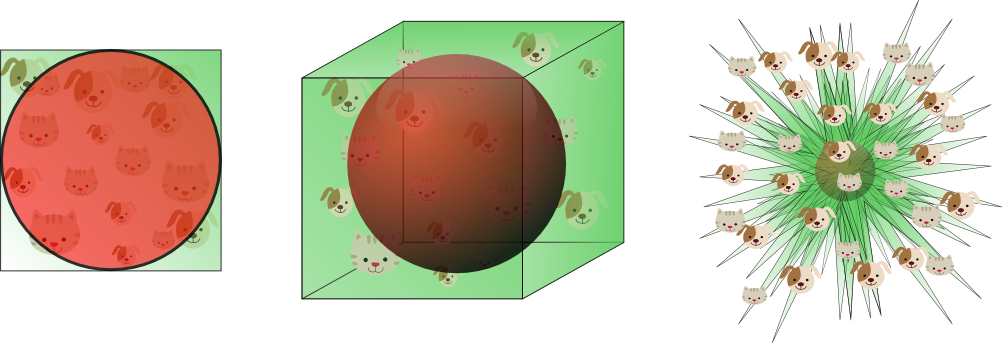# Introduction¶

## 3 Further observations¶

Course: Math 535 - Mathematical Methods in Data Science (MMiDS)
Author: Sebastien Roch, Department of Mathematics, University of Wisconsin-Madison
Updated: Sep 1, 2020

### 3.1 Matrix form of $k$-means clustering¶

The $k$-means clustering objective can be written in matrix form. We will need a notion of matrix norm. A natural way to define a norm for matrices is to notice that an $n \times m$ matrix $A$ can be thought of as an $nm$ vector, with one element for each entry of $A$. Indeed, addition and scalar multiplication work exactly in the same way. Hence, we can define the $2$-norm of a matrix in terms of the sum of its squared entries.

Definition (Frobenius Norm): The Frobenius norm of an $n \times m$ matrix $A \in \mathbb{R}^{n \times m}$ is defined as

$$\|A\|_F = \sqrt{\sum_{i=1}^n \sum_{j=1}^m a_{ij}^2}.$$

$\lhd$

We will encounter other matrix norms later in the course.

As we indicated before, for a collection of $n$ data vectors $\mathbf{x}_1, \ldots, \mathbf{x}_n$ in $\mathbb{R}^d$, it is often convenient to stack them up into a matrix

$$X = \begin{bmatrix} \mathbf{x}_1^T \\ \mathbf{x}_2^T \\ \vdots \\ \mathbf{x}_n^T \\ \end{bmatrix} = \begin{bmatrix} x_{11} & x_{12} & \cdots & x_{1d} \\ x_{21} & x_{22} & \cdots & x_{2d} \\ \vdots & \vdots & \ddots & \vdots \\ x_{n1} & x_{n2} & \cdots & x_{nd} \\ \end{bmatrix}.$$

We can do the same with cluster representatives. Given $\boldsymbol{\mu}_1,\ldots,\boldsymbol{\mu}_k$ also in $\mathbb{R}^d$, we form the matrix

$$U = \begin{bmatrix} \boldsymbol{\mu}_1^T \\ \boldsymbol{\mu}_2^T \\ \vdots \\ \boldsymbol{\mu}_k^T \\ \end{bmatrix} = \begin{bmatrix} \mu_{11} & \mu_{12} & \cdots & \mu_{1d} \\ \mu_{21} & \mu_{22} & \cdots & \mu_{2d} \\ \vdots & \vdots & \ddots & \vdots \\ \mu_{k1} & \mu_{k2} & \cdots & \mu_{kd} \\ \end{bmatrix}.$$

Perhaps less obviously, cluster assignments can also be encoded in matrix form. Recall that, given a partition $C_1,\ldots,C_k$ of $[n]$, we define $c(i) = j$ if $i \in C_j$. For $i=1,\ldots,n$ and $j=1,\ldots,k$, set $z_{ij} = 1$ if $c(i) = j$ and $0$ otherwise, and let $Z$ be the $n \times k$ matrix with entries $z_{ij}$. That is, row $i$ has exactly one entry with value $1$, corresponding to the assigned cluster $c(i)$ of data point $\mathbf{x}_i$, and all other entries $0$.

With this notation, the representative of the cluster assigned to data point $\mathbf{x}_i$ is obtained through the matrix product

$$\boldsymbol{\mu}_{c(i)}^T = \sum_{j = 1}^k z_{ij} \boldsymbol{\mu}_{j}^T = \left(Z U\right)_{i,\cdot}.$$

So

\begin{align*} G(C_1,\ldots,C_k; \boldsymbol{\mu}_1, \ldots, \boldsymbol{\mu}_k) &= \sum_{i=1}^n \|\mathbf{x}_i - \boldsymbol{\mu}_{c(i)}\|^2\\ &= \sum_{i=1}^n \sum_{\ell=1}^d \left(x_{i,\ell} - (Z U)_{i,\ell}\right)^2\\ &= \|X - Z U \|^2_F, \end{align*}

where we used the definition of the Frobenius norm.

In other words, minimizing the $k$-means objective is equivalent to finding a matrix factorization of the form $ZU$ that is a good fit to the data matrix $X$ in Frobenius form. This formulation expresses in a more compact form the idea of representing $X$ as a combination of a small number of representatives. Matrix factorization will come back repeatedly in this course.

### 3.2 High-dimensional space¶

Applying Chebyshev's inequality to sums of independent random variables has useful statistical implications: it shows that, with a large enough number of samples $n$, the sample mean is close to the population mean. Hence it allows us to infer properties of a population from samples. Interestingly, one can apply a similar argument to a different asymptotic regime: the limit of large dimension $d$. But as we will see in this section, the statistical implications are quite different.

#### 3.2.1 High-dimensional cube¶

To start explaining the quote above, we consider a simple experiment. Let $\mathcal{C} = [-1/2,1/2]^d$ be the $d$-cube with side lengths $1$ centered at the origin and let $\mathcal{B} = \{\mathbf{x} \in \mathbb{R}^d : \|x\|\leq 1/2\}$ be the inscribed $d$-ball. In $d=2$ dimensions:(Source)

Now pick a point $\mathbf{X}$ uniformly at random in $\mathcal{C}$. What is the probability that it falls in $\mathcal{B}$?

To generate $\mathbf{X}$, we pick $d$ independent random variables $X_1, \ldots, X_d \sim \mathrm{U}[-1/2, 1/2]$, and form the vector $\mathbf{X} = (X_1, \ldots, X_d)$. Indeed, the PDF of $\mathbf{X}$ is then $f_{\mathbf{X}}(\mathbf{x})= 1^d = 1$ if $\mathbf{x} \in \mathcal{C}$ and $0$ otherwise.

The event we are interested in is $A = \left\{\|\mathbf{X}\| \leq 1/2\right\}$. The uniform distribution over the set $\mathcal{C}$ has the property that $\mathbb{P}[A]$ is the volume of $A$ divided by the volume of $\mathcal{C}$. In this case, the volume of $\mathcal{C}$ is $1^d = 1$ and the volume of $A$ has an explicit formula.

This leads to the following surprising fact:

Theorem (High-dimensional Cube) Let $\mathcal{B} = \{\mathbf{x} \in \mathbb{R}^d \,:\, \|\mathbf{x}\|\leq 1/2\}$ and $\mathcal{C} = [-1/2,1/2]^d$. Pick $\mathbf{X} \sim \mathrm{U}[\mathcal{C}]$. Then, as $d \to +\infty$,

$$\mathbb{P}[\mathbf{X} \in \mathcal{B}] \to 0.$$

In words, in high dimension if one picks a point at random from the cube, it is unlikely to be close to the origin. Instead it is likely to be in the corners. A geometric interpretation is that a high-dimensional cube is a bit like a spiky ball. A visualization of this theorem:(Source)

We give a proof based on Chebyshev's inequality. It has the advantage of providing some insight into this counter-intuitive phenomenon by linking it to the concentration of sums of independent random variables, in this case the squared norm of $\mathbf{X}$.

Proof idea: We think of $\|\mathbf{X}\|^2$ as a sum of independent random variables and apply Chebyshev's inequality. It implies that the norm of $\mathbf{X}$ is concentrated around its mean, which grows like $\sqrt{d}$. The latter is larger than $1/2$ for $d$ large.

Proof: Write out $\|\mathbf{X}\|^2 = \sum_{i=1}^d X_i^2$. Using linearity of expectation and the fact that the $X_i$'s are independent, we get

$$\mathbb{E}\left[ \|\mathbf{X}\|^2 \right] = \sum_{i=1}^d \mathbb{E}[X_i^2] = d \,\mathbb{E}[X_1^2]$$

and

$$\mathrm{Var}\left[ \|\mathbf{X}\|^2 \right] = \sum_{i=1}^d \mathrm{Var}[X_i^2] = d \,\mathrm{Var}[X_1^2].$$

We bound the probability of interest as follows. We first square the norm and center around the mean:

\begin{align} \mathbb{P} \left[ \|\mathbf{X}\| \leq 1/2 \right] &= \mathbb{P} \left[ \|\mathbf{X}\|^2 \leq 1/4 \right]\\ &= \mathbb{P} \left[ \|\mathbf{X}\|^2 - \mathbb{E}[\|\mathbf{X}\|^2]\leq 1/4 - d \,\mathbb{E}[X_1^2] \right]. \end{align}

Now notice that $\mathbb{E}[X_1^2] > 0$ does not depend on $d$. Take $d$ large enough that $d \,\mathbb{E}[X_1^2] > 1/4$. We then use the following fact: if $\alpha = d \mathbb{E}[X_1^2] - 1/4 > 0$ and $Z = \|X\|^2 - \mathbb{E}\|X\|^2$, we can write by monotonicity and the definition of the absolute value

$$\mathbb{P}[Z \leq -\alpha] \leq \mathbb{P}[Z \leq -\alpha\text{ or }Z \geq \alpha] = \mathbb{P}[|Z| \geq \alpha].$$

We arrive at

$$\mathbb{P} \left[ \|\mathbf{X}\| \leq 1/2 \right] \leq \mathbb{P} \left[ \left| \|\mathbf{X}\|^2 - \mathbb{E}[\|\mathbf{X}\|^2] \right| \geq d \,\mathbb{E}[X_1^2] - 1/4 \right].$$

We can now apply Chebyshev's inequality to the right-hand side, which gives

\begin{align} \mathbb{P} \left[ \|\mathbf{X}\| \leq 1/2 \right] &\leq \frac{\mathrm{Var}\left[ \|\mathbf{X}\|^2 \right]} {(d \,\mathbb{E}[X_1^2] - 1/4)^2}\\ &= \frac{d \,\mathrm{Var}[X_1^2]} {(d \,\mathbb{E}[X_1^2] - 1/4)^2}\\ &= \frac{1}{d} \cdot \frac{\mathrm{Var}[X_1^2]} {(\mathbb{E}[X_1^2] - 1/(4d))^2} . \end{align}

Again, $\mathrm{Var}[X_1^2]$ does not depend on $d$. So the right-hand side goes to $0$ as $d \to +\infty$, as claimed.$\square$